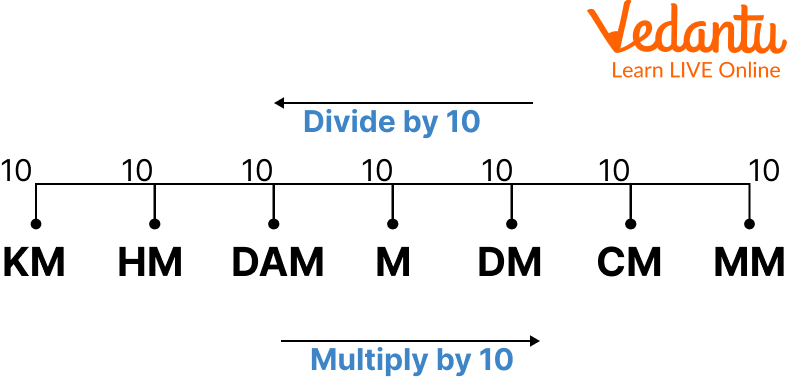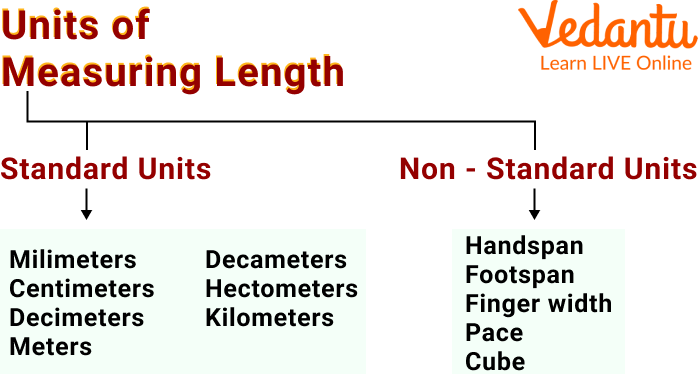Courses
Courses for Kids
Free study material
Free LIVE classes
More

# Measurement Chart of LengthLIVE
Join Vedantu’s FREE Mastercalss

## What is Measurement of Length?

Measuring length involves measuring the length of any object using measuring tools such as a ruler, measuring tape, and so on. A ruler, for example, can be used to measure the length of a pencil in inches. A foot scale can be used to measure the height of students in a class. Inches, centimetres, metres, feet, and more length units exist. The act of measuring the length of things in some specified units, which can be standard or non-standard, is defined as length measurement. Here, we will learn about length units of length, meters conversion chart and will also see the unit conversion chart.

## How to Measure Length?

A ruler has two sides: centimeters/millimeters and inches. The following are the steps for measuring length with a ruler:

Step 1: Select the unit of measurement for length. If you want a value in centimetres, use the ruler's centimetre side. If the measurement is in inches, use the ruler's inches side.

Step 2: Place the object beside the ruler, beginning at point 0.

Step 3: Read the endpoint and record the value.Measure Length

## Units of Length

Length can be measured in several measures such as centimetres, inches, metres, and so on. The length measurement units are divided into two types: Standard length measuring units and non-standard length measuring. Below is the image showing units of measurement chart.Measurement chart

## Standard and Non Standard Units of Measuring Length

Standard units are pre-defined and do not vary from person to person or object to object. If two or more people measure the same object's length in any standard unit, say inches, they will receive the same measurement. Meters, millimetres, inches, feet, yards, are some of the examples of it.

Non standard units do not have a defined numerical measurement. They differ from one person to the next and from one thing to the next. For example, if a youngster and his father use their handspan to measure the length of a rectangular wall, they are likely to get different results. This is because a child's handspan is typically smaller than that of an adult.

## SI Unit of Length

The SI unit of length measurement is the metre (m). The metre is the basic unit of length, as indicated in the measurement chart. Its equivalent in other units is provided below:

• $1 \mathrm{~m}=100 \mathrm{~cm}$

• $1 \mathrm{~m}=1000 \mathrm{~mm}$

• $1 \mathrm{~m}=0.001 \mathrm{~km}$

• $1 \mathrm{~m}=39.37 \text { inches }$

• $1 \mathrm{~m}=1.09361 \text { yards }$

• $1 \mathrm{~m}=3.28 \text { feet }$

## Measurement of Length Chart

The length measurement chart makes it simple to convert one unit to another. For example in the case, if we know that one yard equals 36 inches, we can easily convert three yards to inches by multiplying three by 36.

Let’s see the measurement table in which we will unit conversion chart:

 Metric System 1 cm = 100 mm 1 m = 100 cm 1 m = 1000 mm 1 km = 1000 m Imperial System 1 feet = 12 inches 1 yard = 3 feet 1 yard = 36 inches 1 mile = 1760 yards 1 mile = 5280 feet Metric to Imperial System $1 \mathrm{~cm}=0.3937$ inches $1 \mathrm{~m}=39.37$ inches $1 \mathrm{~m}=3.28$ feet $1 \mathrm{~km}=3280.84$ feet $1 \mathrm{~km}=0.62$ miles $1 \mathrm{~km}=1093.61 \mathrm{yards}$ Imperial to Metric System $1 \mathrm{inch}=2.54 \mathrm{~cm}$ 1 foot $=30.48 \mathrm{~cm}$ $1 \mathrm{yard}=91.44 \mathrm{~cm}$ 1 foot $=0.3048 \mathrm{~m}$ 1 mile $=1.6 \mathrm{~km}$ 1 mile $=1609.34 \mathrm{~m}$ 1 yard $=0.9144 \mathrm{~m}$

## Solved Example

Q 1. $6000 \mathrm{~mL}$ equals how many litres?

Ans: Since, to convert from milliliters to liters, always multiply the number or figure by 0.001 (or divide by 1000).

Here, we have 6000mL given.

So we will divide it by 1000. i.e. $\frac{6000}{1000}$

On solving it, we get

= 6L

Hence, $6000 \mathrm{~mL}$ equals to 6 L.

Q 2. Convert 3 kilometers to meters.

Ans: Since, we know that 1 km = 1000m

Therefore, $3 \mathrm{~km}=3 \mathrm{~km} \times 1000$

$=3000 \mathrm{~m}$

Q 3. Convert $75 \mathrm{~cm}$ to $\mathrm{m}$.

Ans: We know that,

$1 \mathrm{~cm}=0.01 \mathrm{~m}$

For converting $75 \mathrm{~cm}$ to $\mathrm{m}$, multiply $75 \mathrm{~cm}$ by $0.01 \mathrm{~m}$. We get

$75 \mathrm{~cm}=75 \times 0.01 \mathrm{~m}$

$75 \mathrm{~cm}=0.75 \mathrm{~m}$

Thus, $75 \mathrm{~cm}$ is equal to $0.75 \mathrm{~m}$.

## Practice Problem

Q 1. $5000 \mathrm{~mL}$ equals how many litres? (Ans: 5L)

Q 2. Convert 5 cm to m. (Ans: 0.05 m)

Q 3. Convert 210 centimetres to metres. (Ans: 2.1 m)

## Summary

Measurement of length is the act of measuring the length of objects in some specified units which can be standard or non-standard. As we have learned that standard units are pre-defined and it does not vary from person to person. Whereas in the non-standard unit we have seen how the measurement changes as it is initially dependent on the person. Then we learned about the conversion and saw the conversion chart. We hope that this article will give you clarity on the conversion and by solving the practice problem you will have more command over this topic.

Last updated date: 30th Sep 2023
Total views: 112.2k
Views today: 3.12k

## FAQs on Measurement Chart of Length

1. What is the biggest measurement of length?

The biggest unit to measure length is Parsec. It is used to measure space distances. There are approximately 3.26 light-years in 1 Parsec.

2. What is the smallest measurement of length?

The millimetre is the smallest unit of length measurement. We may measure very small objects in millimetres, such as the length of a pencil tip, the length of our fingernail, and so on. A centimetre ruler has 10 tiny divisions between 1 centimetre. Each of these divisions is one millimetre.

3. What are the instruments for measuring length?

There are various instruments or tools for measuring length such as a ruler, yardstick, meter stick, feet scale, measuring tape, etc.